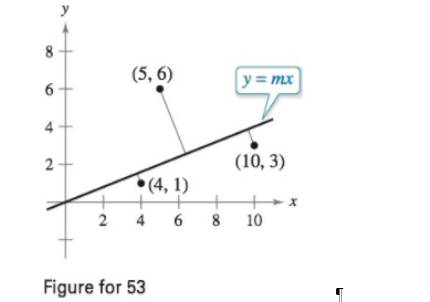Chapter 4.7, Problem 53ECalculus: Early Transcendental Fun...

7th Edition
Ron Larson + 1 other
ISBN: 9781337552516

Solutions

Chapter
SectionCalculus: Early Transcendental Fun...

7th Edition
Ron Larson + 1 other
ISBN: 9781337552516
Textbook Problem

Minimum Distance In Exercises 51-53, consider a fuel distribution center located at the origin of the rectangular coordinate system (units in miles; see figures). The center supplies three factories with coordinates (4,1), (5,6), and(10, 3). A trunk line will run from the distribution center along the line y = m x , and feeder lines will run to the three factories. The objective is to find m such that the lengths of the feeder lines are minimized.Minimize the sum of the lengths of the perpendicular feeder lines (see figure above and Exercise 77 in Section 1.2) from the trunk line to the factories given by S 3 = | 4 m − 1 | m 2 + 1 + | 5 m − 6 | m 2 + 1 + | 10 m − 3 | m 2 + 1 Find the equation of the trunk line by this method and then determine the sum of the lengths of the feeder lines. (Hint: Use a graphing utility to graph the function S3 and approximate the required critical number.)To determine

To calculate: The sum of the length of the feeder lines and the equation of the truck line y=mx such that the distance between the factories and the trick line is minimized.

Explanation

Given:

The distribution centre is located at the origin and the three factories are located at (4,1), (5,6) and (10,3).

The equation for the perpendicular lengths of the feeder lines is:

S3=|4m1|m2+1+|5m6|m2+1+|10m3|m2+1

Formula used:

For a function f that is twice differentiable on an open interval I, if f'(c)=0 for some c, then,

If f''(c)>0 the function f has relative minima at c if f''(c)<0 the function f has relative maxima at c.

Calculation:

This can be done with the help of the Ti-83 calculator.

Step 1: Press the [Y=] key and enter the equation to the right of Y1

Still sussing out bartleby?

Check out a sample textbook solution.

See a sample solution

The Solution to Your Study Problems

Bartleby provides explanations to thousands of textbook problems written by our experts, many with advanced degrees!

Get Started

In Exercises 4756, solve the given equation for the indicated variable. 4=2x

Finite Mathematics and Applied Calculus (MindTap Course List)

In Exercises 3540, rationalize the numerator of each expression. 40. x+3x3

Applied Calculus for the Managerial, Life, and Social Sciences: A Brief Approach

In problems 17-30, simplify each expression so that only positive exponents remain. 29.

Mathematical Applications for the Management, Life, and Social Sciences